# Pythagoras Theorem

Go back to  'Similar-Triangles'

You will already be familiar with the Pythagoras Theorem: in a right-angled triangle, the sum of the squares of the two legs is equal to the square of the hypotenuse, as shown in the figure below: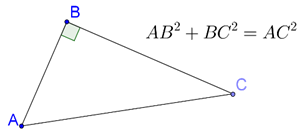We will now prove this theorem using the concept of similarity. For this purpose, draw the perpendicular BD from B onto the hypotenuse AC: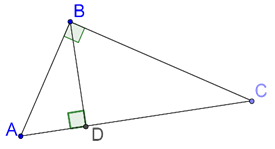Note that you now have two smaller right-angled triangles and one large right-angled triangle. All three are similar to each other, that is,

$$\Delta ABD$$ ~ $$\Delta BCD$$ ~ $$\Delta ACB$$

Can you see how? For example, let us compare $$\Delta ABD$$ and $$\Delta ACB$$:

• $$\angle A$$ = $$\angle A$$ (common)

• $$\angle ADB$$ = $$\angle ABC$$ (right angles)

Thus, the two triangles are equi-angular, which means that they are similar. Carefully notice which vertex in $$\Delta ABD$$ corresponds to which vertex in $$\Delta ACB$$: A corresponds to A, D corresponds to B, and B corresponds to C (respectively).

Similarly, $$\Delta BCD$$ ~ $$\Delta ACB$$ (note carefully the order of the vertices again), and thus all the three triangles are similar.

This means that the ratio of the areas of any two triangles is equal to the ratio of the squares of their corresponding sides. We can write this fact as follows:

$\frac{{ar\Delta(ABC)}}{{A{C^2}}} = \frac{{ar\Delta(ABD)}}{{A{B^2}}} = \frac{{ar\Delta(BCD)}}{{B{C^2}}}$

Now, we use the following basic fact on ratios and proportions:

If

$$\frac{x}{y} = \frac{a}{b},\\ \quad \Rightarrow \frac{x}{y} = \frac{a}{b} = \frac{{x + a}}{{y + b}}$$

Thus,

\begin{align}& \frac{{ar(\Delta ABC)}}{{A{C^2}}} = \frac{{ar(\Delta ABD)}}{{A{B^2}}} = \frac{{ar(\Delta BCD)}}{{B{C^2}}}\\& \Rightarrow \frac{{ar(\Delta ABC)}}{{A{C^2}}} = \frac{{ar(\Delta ABD) + ar(\Delta BCD)}}{{A{B^2} + B{C^2}}} = \frac{{ar(\Delta ABC)}}{{A{B^2} + B{C^2}}}\\& \Rightarrow A{B^2} + B{C^2} = A{C^2} \end{align}

This completes our proof of the Pythagoras Theorem. Another similar proof can be as follows. Since $$\Delta ADB$$ ~ $$\Delta ABC$$, we have:

\begin{align}&\frac{{AD}}{{AB}} = \frac{{AB}}{{AC}}\\& \Rightarrow AD \times AC = A{B^2}\end{align}

Similarly, $$\Delta BDC$$ ~ $$\Delta ABC$$ gives:

\begin{align}&\frac{{CD}}{{BC}} = \frac{{BC}}{{AC}}\\& \Rightarrow CD \times AC = B{C^2}\end{align}

Adding these two relations, we have:

$\begin{array}{l}\left( {AD \times AC + CD \times AC} \right) = A{B^2} + B{C^2}\\ \Rightarrow (AD + CD) \times AC = A{B^2} + B{C^2}\\ \Rightarrow A{C^2} = A{B^2} + B{C^2}\end{array}$

Example 1: N is a point on a straight line segment AB, and $$PN \bot AB$$. Show that

AP2 – BP2 = AN2 – BN2

Solution: Consider the following figure: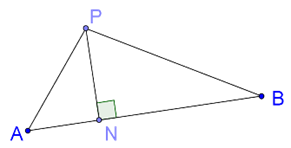By the Pythagoras theorem, we have:

AP2 = AN2 + PN2
BP2 = BN2 + PN2

Subtracting these two relations, we have:

AP2 – BP2 = AN2 – BN2

Example 2: ABC is an equilateral triangle, and AD is the altitude through A. Show that AD2 = 3BD2.

Solution: Consider the following figure: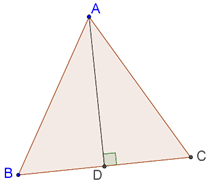Since the triangle is equilateral, the altitude AD is also the median, and so BD = CD = ½(BC). Consider $$\Delta ABD$$. By the Pythagoras theorem, we have:

AB2 = AD2 + BD2

è (2BD)2 = AD2 + BD2

è 4BD2 = AD2 + BD2

è AD2 = 3BD2

Example 3: Prove the converse of the Pythagoras theorem. That is, if in a triangle, the sum of the squares of two sides is equal to the square of the third, show that this triangle is right-angled.

Solution: Consider the following figure: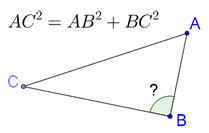It is given that

AC2 = AB2 + BC2

We have to show that $$\Delta ABC$$ is right-angled at B. Let us construct another triangle, $$\Delta DEF$$, such that DE = AB, EF = BC, and $$DE \bot EF$$, as shown below: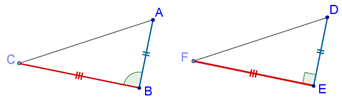By the Pythagoras theorem, DF2 = DE2 + EF2, which means that DF = AC (since DE = AB and EF = BC). Thus, by the SSS criterion, $$\Delta {\rm{ABC }} \equiv {\rm{ }}\Delta {\rm{DEF}}$$, which means that $$\angle B$$ = $$\angle E$$ = 90°.

Example 4: Consider the following figure: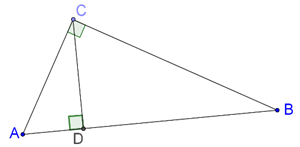It is given that $$\angle ACB$$ = 90°, and  $$CD \bot AB$$. Prove that

$\frac{{B{C^2}}}{{A{C^2}}} = \frac{{BD}}{{AD}}$

Solution: When discussing the proof of the Pythagoras theorem, we saw how the two smaller triangles ($$\Delta ACD$$ and $$\Delta CBD$$) will both be similar to the larger triangle $$\Delta ACB$$ (note the order of the vertices we have used). Thus, $$\Delta ACD$$ ~ $$\Delta ABC$$ gives:

\begin{align}&\frac{{AC}}{{AB}} = \frac{{AD}}{{AC}}\\& \Rightarrow A{C^2} = AB \times AD\end{align}

Similarly, $$\Delta BCD$$ ~ $$\Delta BAC$$ gives

\begin{align}&\frac{{BC}}{{BA}} = \frac{{BD}}{{BC}}\\& \Rightarrow B{C^2} = AB \times BD\end{align}

Dividing the two relations we have obtained, we have:

$\frac{{B{C^2}}}{{A{C^2}}} = \frac{{AB \times BD}}{{AB \times AD}} = \frac{{BD}}{{AD}}$

Example 5: BL and CM are medians of $$\Delta ABC$$ which is right-angled at A. Prove that

$4(B{L^2} + C{M^2}) = 5B{C^2}$

Solution: Consider the following figure: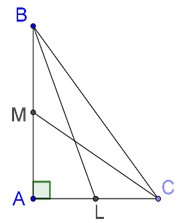We will apply the Pythagoras theorem to $$\Delta ABC$$, $$\Delta ABL$$ and $$\Delta ACM$$. In $$\Delta ABC$$, we have:

$B{C^2} = A{B^2} + A{C^2}$

Now, in $$\Delta ABL$$, we have:

\begin{align}&BL^2=AB^2+AL^2\\&\quad\quad=AB^2+\left(\frac{AC}2\right)^2\\&\Rightarrow 4BL^2=4AB^2+AC^2\;\;\;\;\;\;\;\;\;\;\;\;\cdot\cdot\cdot(1)\end{align}

Next, in $$\Delta ACM$$, we have:

\begin{align}&C{M^2} = A{C^2} + A{M^2}\\&\qquad= A{C^2} + {\left( {\frac{{AB}}{2}} \right)^2}\\& \Rightarrow 4C{M^2} = 4A{C^2} + A{B^2} \;\;\;\;\;\;\;\;\;\;\;\;\cdot \cdot \cdot (2)\end{align}

Finally, we add equations (1) and (2):

\begin{align}&\Rightarrow4(BL^2+CM^2)=\left\{\begin{array}{l}(4AB^2+AC^2)\\\;\;\;\;\;\;\;\;\;\;\;\;+\4AC^2+AB^2)\end{array}\right.\\&\Rightarrow4(BL^2+CM^2)=5(AB^2+AC^2)=5BC^2\end{align} Example 6: O is an arbitrary point inside a rectangle ABCD. Show that $O{A^2} + O{C^2} = O{B^2} + O{D^2}$ Solution: Consider the following figure: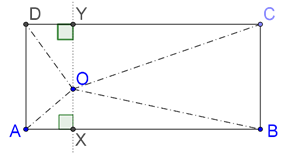Through O, we have drawn XY || BC, intersecting AB at X and CD at Y. Clearly, \(OX \bot AB and $$OY \bot CD$$ . Now, we will make use of the Pythagoras theorem, as follows:

\begin{align}&OA^2+OC^2=OX^2+AX^2+OY^2+CY^2\\&\qquad\qquad\quad=OX^2+DY^2+OY^2+BX^2\;\;\;\;\;\;(how?)\\&\qquad\qquad\quad=(OX^2+BX^2)+(OY^2+DY^2)\\&\qquad\qquad\quad=OB^2+OD^2\end{align}

Learn math from the experts and clarify doubts instantly

• Instant doubt clearing (live one on one)
• Learn from India’s best math teachers
• Completely personalized curriculum